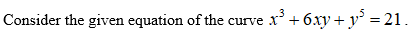# Find an equation of the line tangent to the curve defined byx^3+6xy+y^5=21 at the point (2,1)

Question
3 views

Find an equation of the line tangent to the curve defined by

x^3+6xy+y^5=21 at the point (2,1)

check_circle

Step 1Step 2

Differentiate the given equation with respect to x.

Step 3

Now substitute the given point (2, 1) in the above obtained ...

### Want to see the full answer?

See Solution

#### Want to see this answer and more?

Solutions are written by subject experts who are available 24/7. Questions are typically answered within 1 hour.*

See Solution
*Response times may vary by subject and question.
Tagged in

### Other